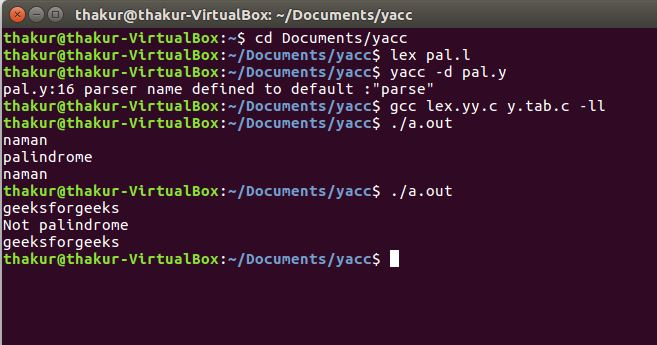Related Articles

# YACC program to check whether given string is Palindrome or not

• Difficulty Level : Medium
• Last Updated : 15 Oct, 2019

Problem: Write a YACC program to check whether given string is Palindrome or not.

Explanation:
Yacc (for “yet another compiler compiler.”) is the standard parser generator for the Unix operating system. An open source program, yacc generates code for the parser in the C programming language. The acronym is usually rendered in lowercase but is occasionally seen as YACC or Yacc.

Examples:

```Input: naman
Output: palindrome

Input: geeksforgeeks
Output: not palindrome ```

Lexical Analyzer Source Code :

 `%{``    ``/* Definition section */``    ``#include ``    ``#include ``    ``#include "y.tab.h"``%}`` ` `/* %option noyywrap */`` ` `/* Rule Section */``%%`` ` `[a-zA-Z]+   {yylval.f = yytext; ``return` `STR;}``[-+()*/]    {``return` `yytext;}``[ \t\n]      {;}`` ` `%%`` ` ` ``int` `yywrap()`` ``{ ``  ``return` `-1; `` ``}  `

Parser Source Code:

 `%{``    ``/* Definition section */``    ``#include ``    ``#include    ``    ``#include ``    ``extern` `int` `yylex();``    ` `    ``void` `yyerror(``char` `*msg);``    ``int` `flag;``    ` `    ``int` `i;``    ``int` `k =0;       ``%}`` ` `%``union` `{``    ``char``* f;`` ``}`` ` `%token STR``%type E`` ` `/* Rule Section */``%%`` ` `S : E    {``         ``flag = 0;``         ``k = ``strlen``(\$1) - 1;``         ``if``(k%2==0){   ``          ` `         ``for` `(i = 0; i <= k/2; i++) {``           ``if` `(\$1[i] == \$1[k-i]) {``            ``} ``else` `{``               ``flag = 1;``              ``}``          ``}``         ``if` `(flag == 1) ``printf``(``"Not palindrome\n"``);``         ``else` `printf``(``"palindrome\n"``);``         ``printf``(``"%s\n"``, \$1);``          ` `        ``}``else``{``         ` `        ``for` `(i = 0; i < k/2; i++) {``          ``if` `(\$1[i] == \$1[k-i]) {``          ``} ``else` `{``              ``flag = 1;``             ``}``            ``}``        ``if` `(flag == 1) ``printf``(``"Not palindrome\n"``);``        ``else` `printf``(``"palindrome\n"``);``        ``printf``(``"%s\n"``, \$1);           ``        ` ` ` `          ``}``       ``}``  ``;`` ` `E :  STR    {\$\$ = \$1;}``  ``;`` ` `%%`` ` `void` `yyerror(``char` `*msg)`` ``{``    ``fprintf``(stderr, ``"%s\n"``, msg);``    ``exit``(1);`` ``}`` ` `//driver code ``int` `main()`` ``{``    ``yyparse();``    ``return` `0;`` ``}`

Output:Attention reader! Don’t stop learning now. Get hold of all the important CS Theory concepts for SDE interviews with the CS Theory Course at a student-friendly price and become industry ready.

My Personal Notes arrow_drop_up